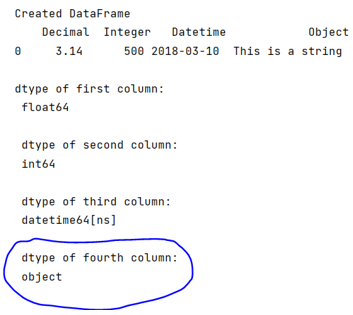# What is dtype('O') in Pandas?

Learn, what is dtype('O') in Pandas?
Submitted by Pranit Sharma, on June 18, 2022

Pandas is a special tool that allows us to perform complex manipulations of data effectively and efficiently. Inside pandas, we mostly deal with a dataset in the form of DataFrame. DataFrames are 2-dimensional data structures in pandas. DataFrames consist of rows, columns, and the data.

## Problem statement

Sometimes we need to check each and every data type of the columns of a pandas DataFrame.

## dtype('O') in Pandas

For this purpose, we use pandas.DataFrame.dtypes property. As a result, we sometimes get a dtype as 'O'.

'O' means python object. And pandas object is nothing but string. If any string is encountered while pandas.DataFrame.dtypes checks for dtypes of all the values, and it returns the dtype 'O' i.e., an object.

To work with pandas, we need to import pandas package first, below is the syntax:

```import pandas as pd
```

Let us understand with the help of an example,

## Python program to demonstrate the use of dtype('O') in Pandas

```# Importing pandas package
import pandas as pd

# Creating a DataFrame
df = pd.DataFrame({
'Decimal': [3.14],
'Integer': ,
'Datetime': [pd.Timestamp('20180310')],
'Object': ['This is a string']
})

# Display DataFrame
print("Created DataFrame\n",df,"\n")

# Display all the dtypes
print("dtype of first column:\n",
df['Decimal'].dtype,"\n\n",
"dtype of second column:\n",
df['Integer'].dtype,
"\n\n",
"dtype of third column:\n",
df['Datetime'].dtype,
"\n\n",
"dtype of fourth column:\n",
df['Object'].dtype)
```

## Output

The output of the above program is: